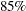# The QUANTLIFE Procedure

## Overview: QUANTLIFE Procedure

Subsections:

The QUANTLIFE procedure performs quantile regression analysis for survival data, in which observations are not always directly observed.

Quantile regression analysis (Koenker and Bassett, 1978) is a type of regression analysis that explores how the conditional quantile of a response variable depends on its covariates. Recall that a student’s score on a test is at the 0.85th quantile if his or her score is higher than that ofof the students who took the test. The score is also said to be at the 85th percentile. By estimating a set of conditional quantiles, you can gain more insight into the conditional distribution of the response, given its covariates.

Quantile regression provides a flexible way to capture heterogeneous effects in the sense that the tails and the central location of the conditional distributions can vary differently with the covariates. Thus, quantile regression offers a powerful tool in survival analysis, where the lifetimes are skewed and extreme survival times can be of special interest (Koenker and Geling, 2001; Huang, 2010).

When the observations are fully observed, you can use the QUANTREG procedure to fit a standard quantile regression model. For an introduction to the basic concepts of quantile regression analysis, see Chapter 83: The QUANTREG Procedure.

However, lifetime data often contain incomplete observations because of censoring (Klein and Moeschberger, 2003; Hosmer, Lemeshow, and May, 2008). When censoring occurs, the usual standard quantile regression approach can lead to biased estimates. Thus, special approaches have been developed that account for censoring and provide valid estimates. Portnoy (2003) proposed a method to estimate conditional quantile functions by generalizing the idea of the Kaplan-Meier estimator of the survival function. Peng and Huang (2008) developed a different quantile regression approach that is motivated by the Nelson-Aalen estimator of the cumulative hazard function. Both methods can be implemented using linear programming algorithms, and both are available in the QUANTLIFE procedure. Like the standard quantile regression method for uncensored data, these two methods are distribution-free and apply to heteroscedastic data.